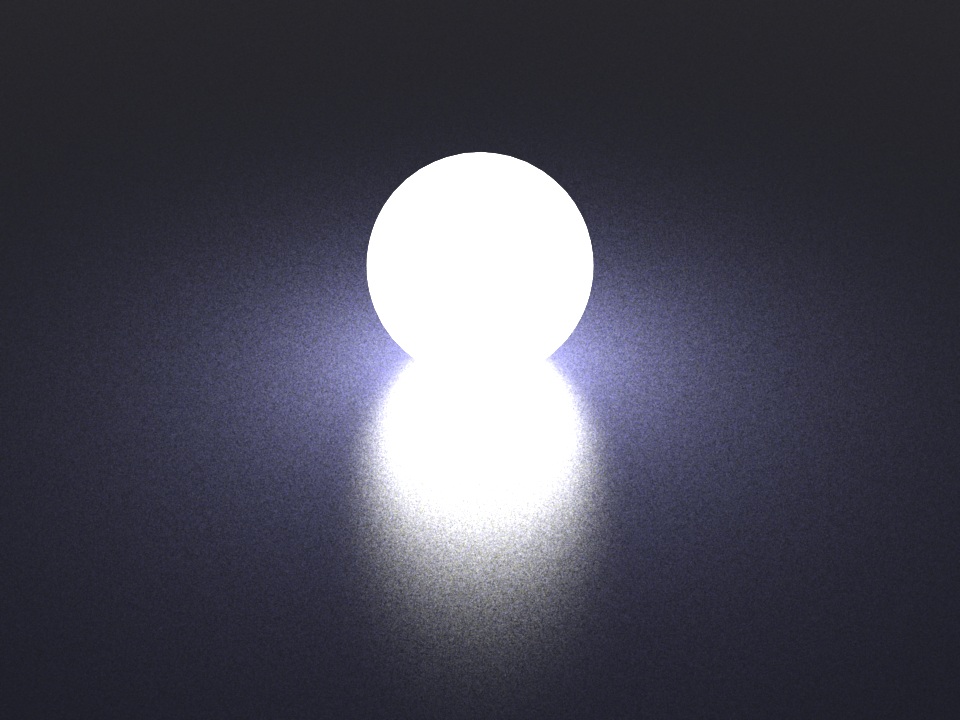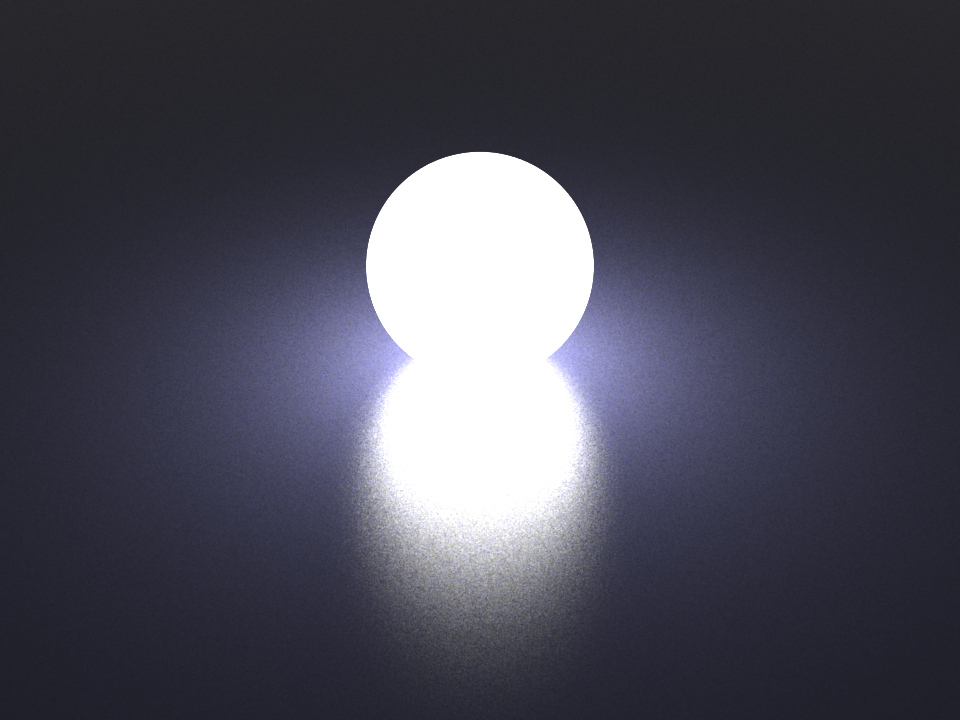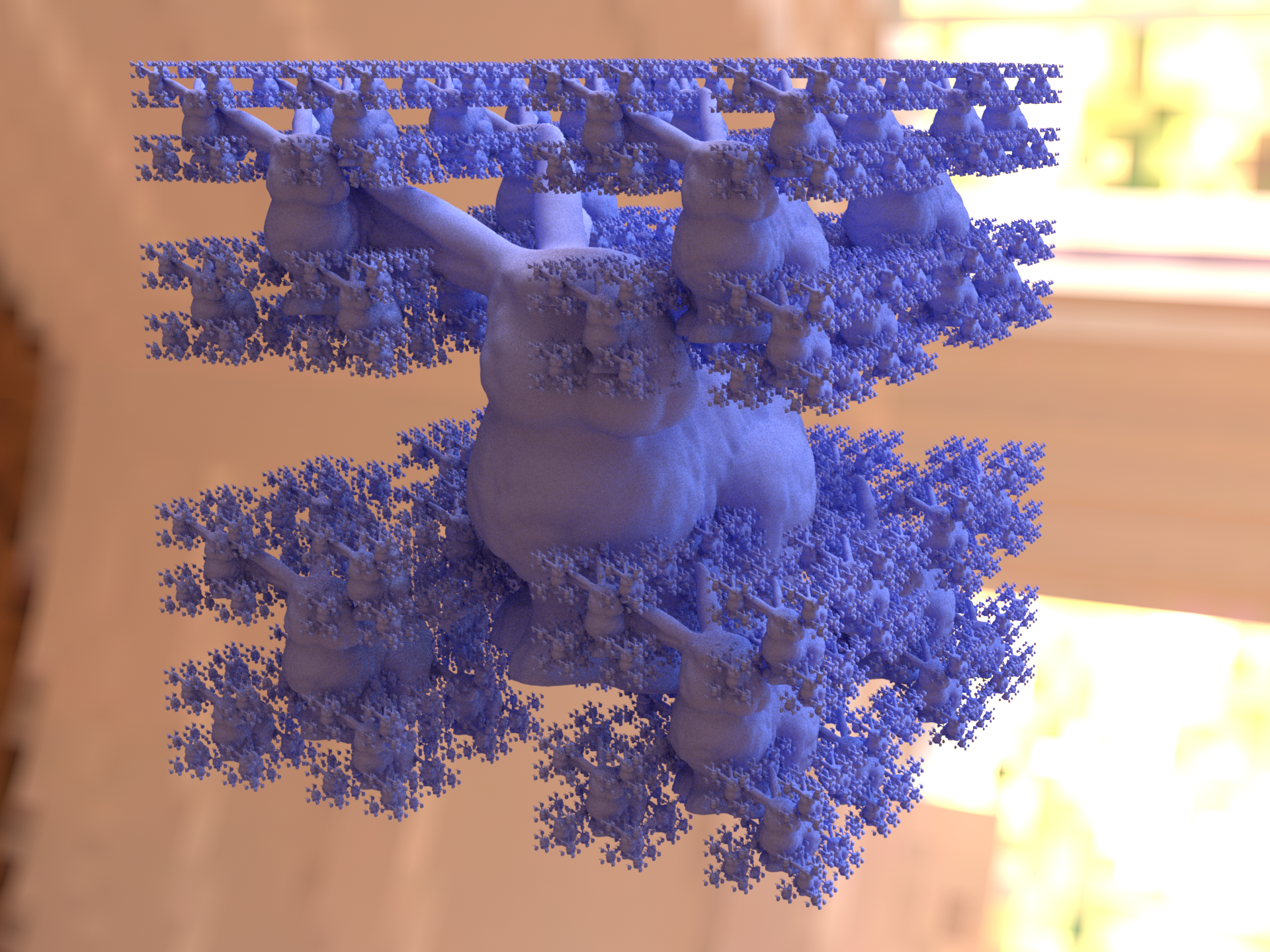# Shapes#

This section presents an overview of the shape plugins that are released along with the renderer.

In Mitsuba 3, shapes define surfaces that mark transitions between different types of materials. For instance, a shape could describe a boundary between air and a solid object, such as a piece of rock. Alternatively, a shape can mark the beginning of a region of space that isn’t solid at all, but rather contains a participating medium, such as smoke or steam. Finally, a shape can be used to create an object that emits light on its own.

Shapes are usually declared along with a surface scattering model named BSDF (see the respective section). This BSDF characterizes what happens at the surface. In the XML scene description language, this might look like the following:

<scene version=3.0.0>
<!-- .. scene contents .. -->

<shape type=".. shape type ..">
.. shape parameters ..

<bsdf type=".. BSDF type ..">
.. bsdf parameters ..
</bsdf>

<!-- Alternatively: reference a named BSDF that
has been declared previously

<ref id="my_bsdf"/>
-->
</shape>
</scene>


The following subsections discuss the available shape types in greater detail.

## Wavefront OBJ mesh loader (obj)#

Parameter

Type

Description

Flags

filename

string

Filename of the OBJ file that should be loaded

face_normals

boolean

When set to true, any existing or computed vertex normals are discarded and face normals will instead be used during rendering. This gives the rendered object a faceted appearance. (Default: false)

flip_tex_coords

boolean

Treat the vertical component of the texture as inverted? Most OBJ files use this convention. (Default: true)

flip_normals

boolean

Is the mesh inverted, i.e. should the normal vectors be flipped? (Default:false, i.e. the normals point outside)

vertex_count

integer

Total number of vertices

P

State parameters

face_count

integer

Total number of faces

P

faces

uint32[]

Face indices buffer (flatten)

P

vertex_positions

float[]

Vertex positions buffer (flatten) pre-multiplied by the object-to-world transformation.

P, , D

vertex_normals

float[]

Vertex normals buffer (flatten) pre-multiplied by the object-to-world transformation.

P, , D

vertex_texcoords

float[]

Vertex texcoords buffer (flatten)

P,

(Mesh attribute)

float[]

Mesh attribute buffer (flatten)

P,

to_world

transform

Specifies an optional linear object-to-world transformation. (Default: none, i.e. object space = world space)

This plugin implements a simple loader for Wavefront OBJ files. It handles meshes containing triangles and quadrilaterals, and it also imports vertex normals and texture coordinates.

Loading an ordinary OBJ file is as simple as writing:

<shape type="obj">
<string name="filename" value="my_shape.obj"/>
</shape>


Note

Importing geometry via OBJ files should only be used as an absolutely last resort. Due to inherent limitations of this format, the files tend to be unreasonably large, and parsing them requires significant amounts of memory and processing power. What’s worse is that the internally stored data is often truncated, causing a loss of precision. If possible, use the ply or serialized plugins instead.

## PLY (Stanford Triangle Format) mesh loader (ply)#

Parameter

Type

Description

Flags

filename

string

Filename of the PLY file that should be loaded

face_normals

boolean

When set to true, any existing or computed vertex normals are discarded and face normals will instead be used during rendering. This gives the rendered object a faceted appearance. (Default: false)

flip_normals

boolean

Is the mesh inverted, i.e. should the normal vectors be flipped? (Default:false, i.e. the normals point outside)

to_world

transform

Specifies an optional linear object-to-world transformation. (Default: none, i.e. object space = world space)

State parameters

vertex_count

integer

Total number of vertices

P

face_count

integer

Total number of faces

P

faces

uint32[]

Face indices buffer (flatten)

P

vertex_positions

float[]

Vertex positions buffer (flatten) pre-multiplied by the object-to-world transformation.

P, , D

vertex_normals

float[]

Vertex normals buffer (flatten) pre-multiplied by the object-to-world transformation.

P, , D

vertex_texcoords

float[]

Vertex texcoords buffer (flatten)

P,

(Mesh attribute)

float[]

Mesh attribute buffer (flatten)

P,

This plugin implements a fast loader for the Stanford PLY format (both the ASCII and binary format, which is preferred for performance reasons). The current plugin implementation supports triangle meshes with optional UV coordinates, vertex normals and other custom vertex or face attributes.

Consecutive attributes with names sharing a common prefix and using one of the following schemes:

{prefix}_{x|y|z|w}, {prefix}_{r|g|b|a}, {prefix}_{0|1|2|3}, {prefix}_{1|2|3|4}

will be group together under a single multidimensional attribute named {vertex|face}_{prefix}.

RGB color attributes can also be defined without a prefix, following the naming scheme {r|g|b|a} or {red|green|blue|alpha}. Those attributes will be group together under a single multidimensional attribute named {vertex|face}_color.

<shape type="ply">
<string name="filename" value="my_shape.ply"/>
<boolean name="flip_normals" value="true"/>
</shape>


Note

Values stored in a RBG color attribute will automatically be converted into spectral model coefficients when using a spectral variant of the renderer.

## Serialized mesh loader (serialized)#

Parameter

Type

Description

Flags

filename

string

Filename of the OBJ file that should be loaded

shape_index

integer

A .serialized file may contain several separate meshes. This parameter specifies which one should be loaded. (Default: 0, i.e. the first one)

face_normals

boolean

When set to true, any existing or computed vertex normals are discarded and emph{face normals} will instead be used during rendering. This gives the rendered object a faceted appearance. (Default: false)

flip_normals

boolean

Is the mesh inverted, i.e. should the normal vectors be flipped? (Default:false, i.e. the normals point outside)

to_world

transform

Specifies an optional linear object-to-world transformation. (Default: none, i.e. object space = world space)

State parameters

vertex_count

integer

Total number of vertices

P

face_count

integer

Total number of faces

P

faces

uint32[]

Face indices buffer (flatten)

P

vertex_positions

float[]

Vertex positions buffer (flatten) pre-multiplied by the object-to-world transformation.

P, , D

vertex_normals

float[]

Vertex normals buffer (flatten) pre-multiplied by the object-to-world transformation.

P, , D

vertex_texcoords

float[]

Vertex texcoords buffer (flatten)

P,

(Mesh attribute)

float[]

Mesh attribute buffer (flatten)

P,

The serialized mesh format represents the most space and time-efficient way of getting geometry information into Mitsuba 3. It stores indexed triangle meshes in a lossless gzip-based encoding that (after decompression) nicely matches up with the internally used data structures. Loading such files is considerably faster than the ply plugin and orders of magnitude faster than the obj plugin.

### Format description#

The serialized file format uses the little endian encoding, hence all fields below should be interpreted accordingly. The contents are structured as follows:

Type

Content

uint16

File format identifier: 0x041C

uint16

File version identifier. Currently set to 0x0004

$$\rightarrow$$

From this point on, the stream is compressed by the DEFLATE algorithm.

$$\rightarrow$$

The used encoding is that of the zlib library.

uint32

An 32-bit integer whose bits can be used to specify the following flags:

• 0x0001: The mesh data includes per-vertex normals

• 0x0002: The mesh data includes texture coordinates

• 0x0008: The mesh data includes vertex colors

• 0x0010: Use face normals instead of smoothly interpolated vertex normals. Equivalent to specifying face_normals=true to the plugin.

• 0x1000: The subsequent content is represented in single precision

• 0x2000: The subsequent content is represented in double precision

string

A null-terminated string (utf-8), which denotes the name of the shape.

uint64

Number of vertices in the mesh

uint64

Number of triangles in the mesh

array

Array of all vertex positions (X, Y, Z, X, Y, Z, …) specified in binary single or double precision format (as denoted by the flags)

array

Array of all vertex normal directions (X, Y, Z, X, Y, Z, …) specified in binary single or double precision format. When the mesh has no vertex normals, this field is omitted.

array

Array of all vertex texture coordinates (U, V, U, V, …) specified in binary single or double precision format. When the mesh has no texture coordinates, this field is omitted.

array

Array of all vertex colors (R, G, B, R, G, B, …) specified in binary single or double precision format. When the mesh has no vertex colors, this field is omitted.

array

Indexed triangle data ([i1, i2, i3], [i1, i2, i3], ..) specified in uint32 or in uint64 format (the latter is used when the number of vertices exceeds 0xFFFFFFFF).

### Multiple shapes#

It is possible to store multiple meshes in a single .serialized file. This is done by simply concatenating their data streams, where every one is structured according to the above description. Hence, after each mesh, the stream briefly reverts back to an uncompressed format, followed by an uncompressed header, and so on. This is necessary for efficient read access to arbitrary sub-meshes.

### End-of-file dictionary#

In addition to the previous table, a .serialized file also concludes with a brief summary at the end of the file, which specifies the starting position of each sub-mesh:

Type

Content

uint64

File offset of the first mesh (in bytes)—this is always zero.

uint64

File offset of the second mesh

$$\cdots$$

$$\cdots$$

uint64

File offset of the last sub-shape

uint32

Total number of meshes in the .serialized file

<shape type="serialized">
<string name="filename" value="shape.serialized"/>
<bsdf type='diffuse'/>
</shape>


## Sphere (sphere)#

Parameter

Type

Description

Flags

center

point

Center of the sphere (Default: (0, 0, 0))

float

Radius of the sphere (Default: 1)

flip_normals

boolean

Is the sphere inverted, i.e. should the normal vectors be flipped? (Default:false, i.e. the normals point outside)

to_world

transform

Specifies an optional linear object-to-world transformation. Note that non-uniform scales and shears are not permitted! (Default: none, i.e. object space = world space)

This shape plugin describes a simple sphere intersection primitive. It should always be preferred over sphere approximations modeled using triangles.

A sphere can either be configured using a linear to_world transformation or the center and radius parameters (or both). The two declarations below are equivalent.

<shape type="sphere">
<transform name="to_world">
<scale value="2"/>
<translate x="1" y="0" z="0"/>
</transform>
<bsdf type="diffuse"/>
</shape>

<shape type="sphere">
<point name="center" x="1" y="0" z="0"/>
<bsdf type="diffuse"/>
</shape>


When a sphere shape is turned into an area light source, Mitsuba 3 switches to an efficient sampling strategy by Fred Akalin that has particularly low variance. This makes it a good default choice for lighting new scenes.Spherical area light modeled using triangles#Spherical area light modeled using the sphere plugin#

## Cylinder (cylinder)#

Parameter

Type

Description

Flags

p0

point

Object-space starting point of the cylinder’s centerline. (Default: (0, 0, 0))

p1

point

Object-space endpoint of the cylinder’s centerline (Default: (0, 0, 1))

float

Radius of the cylinder in object-space units (Default: 1)

flip_normals

boolean

Is the cylinder inverted, i.e. should the normal vectors be flipped? (Default: false, i.e. the normals point outside)

to_world

transform

Specifies an optional linear object-to-world transformation. Note that non-uniform scales are not permitted! (Default: none, i.e. object space = world space)

P

This shape plugin describes a simple cylinder intersection primitive. It should always be preferred over approximations modeled using triangles. Note that the cylinder does not have endcaps – also, its normals point outward, which means that the inside will be treated as fully absorbing by most material models. If this is not desirable, consider using the twosided plugin.

A simple example for instantiating a cylinder, whose interior is visible:

<shape type="cylinder">
<bsdf type="twosided">
<bsdf type="diffuse"/>
</bsdf>
</shape>


## Disk (disk)#

Parameter

Type

Description

Flags

flip_normals

boolean

Is the disk inverted, i.e. should the normal vectors be flipped? (Default: false)

to_world

transform

Specifies a linear object-to-world transformation. Note that non-uniform scales are not permitted! (Default: none, i.e. object space = world space)

P

This shape plugin describes a simple disk intersection primitive. It is usually preferable over discrete approximations made from triangles.

By default, the disk has unit radius and is located at the origin. Its surface normal points into the positive Z-direction. To change the disk scale, rotation, or translation, use the to_world parameter.

The following XML snippet instantiates an example of a textured disk shape:

<shape type="disk">
<bsdf type="diffuse">
<texture name="reflectance" type="checkerboard">
<transform name="to_uv">
<scale x="2" y="10" />
</transform>
</texture>
</bsdf>
</shape>


## Rectangle (rectangle)#

Parameter

Type

Description

Flags

flip_normals

boolean

Is the rectangle inverted, i.e. should the normal vectors be flipped? (Default: false)

to_world

transform

Specifies a linear object-to-world transformation. (Default: none (i.e. object space = world space))

P

This shape plugin describes a simple rectangular shape primitive. It is mainly provided as a convenience for those cases when creating and loading an external mesh with two triangles is simply too tedious, e.g. when an area light source or a simple ground plane are needed. By default, the rectangle covers the XY-range $$[-1,1]\times[-1,1]$$ and has a surface normal that points into the positive Z-direction. To change the rectangle scale, rotation, or translation, use the to_world parameter.

The following XML snippet showcases a simple example of a textured rectangle:

<shape type="rectangle">
<bsdf type="diffuse">
<texture name="reflectance" type="checkerboard">
<transform name="to_uv">
<scale x="5" y="5" />
</transform>
</texture>
</bsdf>
</shape>


## Shape group (shapegroup)#

Parameter

Type

Description

Flags

(Nested plugin)

shape

One or more shapes that should be made available for geometry instancing

This plugin implements a container for shapes that should be made available for geometry instancing. Any shapes placed in a shapegroup will not be visible on their own—instead, the renderer will precompute ray intersection acceleration data structures so that they can efficiently be referenced many times using the Instance (instance) plugin. This is useful for rendering things like forests, where only a few distinct types of trees have to be kept in memory. An example is given below:

<!-- Declare a named shape group containing two objects -->
<shape type="shapegroup" id="my_shape_group">
<shape type="ply">
<string name="filename" value="data.ply"/>
<bsdf type="roughconductor"/>
</shape>
<shape type="sphere">
<transform name="to_world">
<scale value="5"/>
<translate y="20"/>
</transform>
<bsdf type="diffuse"/>
</shape>
</shape>

<!-- Instantiate the shape group without any kind of transformation -->
<shape type="instance">
<ref id="my_shape_group"/>
</shape>

<!-- Create instance of the shape group, but rotated, scaled, and translated -->
<shape type="instance">
<ref id="my_shape_group"/>
<transform name="to_world">
<rotate x="1" angle="45"/>
<scale value="1.5"/>
<translate z="10"/>
</transform>
</shape>


## Instance (instance)#

Parameter

Type

Description

Flags

(Nested plugin)

shapegroup

A reference to a shape group that should be instantiated.

to_world

transform

Specifies a linear object-to-world transformation. (Default: none (i.e. object space = world space))

This plugin implements a geometry instance used to efficiently replicate geometry many times. For details on how to create instances, refer to the Shape group (shapegroup) plugin.The Stanford bunny loaded a single time and instanciated 1365 times (equivalent to 100 million triangles)

Warning

• Note that it is not possible to assign a different material to each instance — the material assignment specified within the shape group is the one that matters.

• Shape groups cannot be used to replicate shapes with attached emitters, sensors, or subsurface scattering models.

## Cube (cube)#

Parameter

Type

Description

Flags

flip_normals

boolean

Is the cube inverted, i.e. should the normal vectors be flipped? (Default:false, i.e. the normals point outside)

vertex_count

integer

Total number of vertices

P

face_count

integer

Total number of faces

P

faces

uint32[]

Face indices buffer (flatten)

P

vertex_positions

float[]

Vertex positions buffer (flatten) pre-multiplied by the object-to-world transformation.

P, , D

vertex_normals

float[]

Vertex normals buffer (flatten) pre-multiplied by the object-to-world transformation.

P, , D

vertex_texcoords

float[]

Vertex texcoords buffer (flatten)

P,

(Mesh attribute)

float[]

Mesh attribute buffer (flatten)

P,

(Mesh attribute)

float[]

Mesh attribute buffer (flatten)

P,

to_world

transform

Specifies an optional linear object-to-world transformation. (Default: none (i.e. object space = world space))

This shape plugin describes a cube intersection primitive, based on the triangle mesh class. By default, it creates a cube between the world-space positions (−1, −1, −1) and (1, 1, 1). However, an arbitrary linear transformation may be specified to translate, rotate, scale or skew it as desired. The parameterization of this shape maps every face onto the rectangle $$[0, 1]^2$$ in uv space.

<shape type="cube">
<transform name="to_world">
<scale x="2" y="10" z="1"/>
</transform>
</shape>